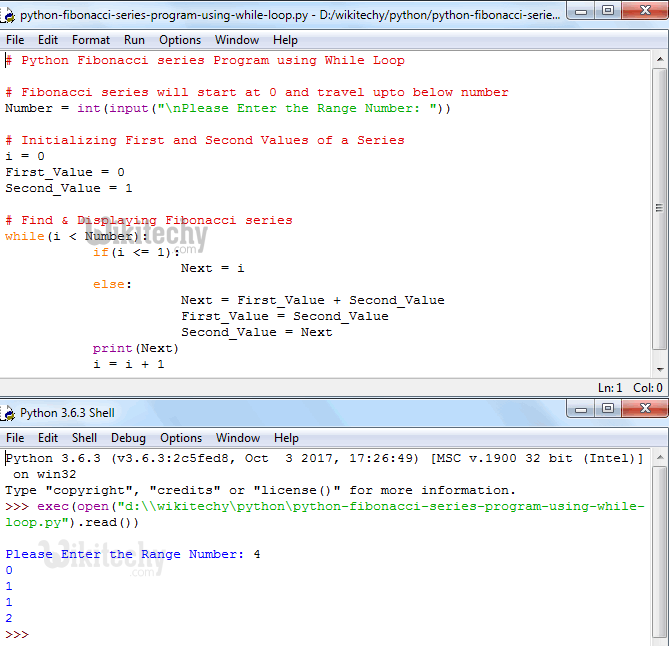# python tutorial - Python Fibonacci Series program - learn python - python programming

## Fibonacci Series

• In Mathematics, Fibonacci Series or Fibonacci Numbers are the numbers that are displayed in following sequence.
• Fibonacci Series = 0, 1, 1, 2, 3, 5, 8, 13, 21, 34 …
• If you observe the above pattern, First Value is 0, Second Value is 1 and the subsequent number is the result of sum of the previous two numbers. For example, Third value is (0 + 1), Fourth value is (1 + 1) so on and so forth.
• In this article we will show you, How to Write Python Fibonacci Series program using While Loop, For Loop and Recursion.Learn Python - Python tutorial - Python Fibonacci Series program - Python examples - Python programs

## Python Fibonacci Series program Using While Loop

• This Python program allows the user to enter any positive integer and then, this program will display the fibonacci series of number from 0 to user specified number using the Python While Loop

## OUTPUTLearn Python - Python tutorial - Python Fibonacci Series program Using While Loop - Python examples - Python programs

## ANALYSIS

• This program allows the user to enter any positive integer and then, that number is assigned to variable Number. Next, We declared three integer variables i, First_Value and Second_Value and assigned values as we shown above. Below While loop will make sure that, the loop will start from 0 and it is less than the user given number. Within the While loop, we used If statement.
• If i value is less than or equal to 1 then, Next will be i
• If i value is greater than 1, perform calculations inside the Else block.
• Let us see the working principle of this while loop in iteration wise.
• User Entered value: Number = 4 and i = 0, First_Value = 0, Second_Value = 1

## First Iteration

• While (0 < 4) is TRUE so, program will start executing statements inside the while loop
• Within the while loop we have If statement and the condition if (0 <= 1) is TRUE so Next = 0 and compiler will exit from if statement block
• Print statement print(Next) will print the value 0
• Lastly, i will be incremented to 1

## Second Iteration

• While (1 < 4) is TRUE so, program will start executing statements inside the while loop
• Within the while loop we have If statement and the condition if (1 <= 1) is TRUE so Next = 1 and compiler will exit from if statement block
• Print statement print(Next) will print the value 1
• Lastly, i will be incremented to 1

## Third Iteration

• While (2 < 4) is TRUE so, program will start executing statements inside the while loop. Within the while loop we have If statement and the condition if (2 <= 1) is FALSE so statements inside the else block will start executing
• Next = First_Value + Second_Value
• Next = 0 + 1 = 1
• First_Value = Second_Value = 1
• Second_Value = Next = 1
• Next, Print statement print(Next) will print the value 1. Lastly, i will be incremented to 1

## Fourth Iteration

• While (3 < 4) is TRUE so, program will start executing statements inside the while loop. Within the while loop we have If statement and the condition if (3 <= 1) is FALSE so statements inside the else block will start executing
• Next = First_Value + Second_Value
• Next = 1 + 1 = 2
• First_Value = Second_Value = 1
• Second_Value = Next = 2
• Next, Print statement print(Next) will print the value 2. Lastly, i will be incremented to 1

## Fifth Iteration

• While (4 < 4) is FALSE so, program will exit from the while loop.
• From the above, Our final output of Next values are: 0 1 1 2

## Python Fibonacci Series program Using For Loop

• This Python program allows the user to enter any positive integer and then, this program will display the fibonacci series of number from 0 to user specified number using Python For Loop

## OUTPUTLearn Python - Python tutorial - Python Fibonacci Series program Using For Loop - Python examples - Python programs

• We just replaced the While loop in the above example with the For loop. If you don’t understand the for loop then please refer For Loop article here: Python For Loop

## Python Fibonacci Series program Using Recursion

• This Python program allows the user to enter any positive integer and then, this program will display the fibonacci series of number from 0 to user specified number using Recursion concept.

## OUTPUTLearn Python - Python tutorial - Python Fibonacci Series program Using Recursion - Python examples - Python programs

## ANALYSIS

• In this example we defined a function. Following function will accept integer values as parameter value and return value
• if (Number == 0) will check whether the given number is 0 or not. If it is TRUE, function will return the value Zero.
• if (Number == 1) will check whether the given number is 1 or not. If it is TRUE, function will return the value One.
• If the number is greater than 1 then the statements inside the else block will be executed.
• Within the Else block we called the function recursively to display the Fibonacci series.

For example, Number = 2
(Fibonacci_series(Number – 2)+ Fibonacci_series(Number – 1))
(Fibonacci_series(2 – 2)+ Fibonacci_series(2 – 1)), It means
(Fibonacci_series(0)+ Fibonacci_series(1))
return (0 + 1) = return 1

• NOTE: For Recursive functions it is very important to place a condition before using the function recursively otherwise, we will end up in infinite execution (Same like infinite Loop).